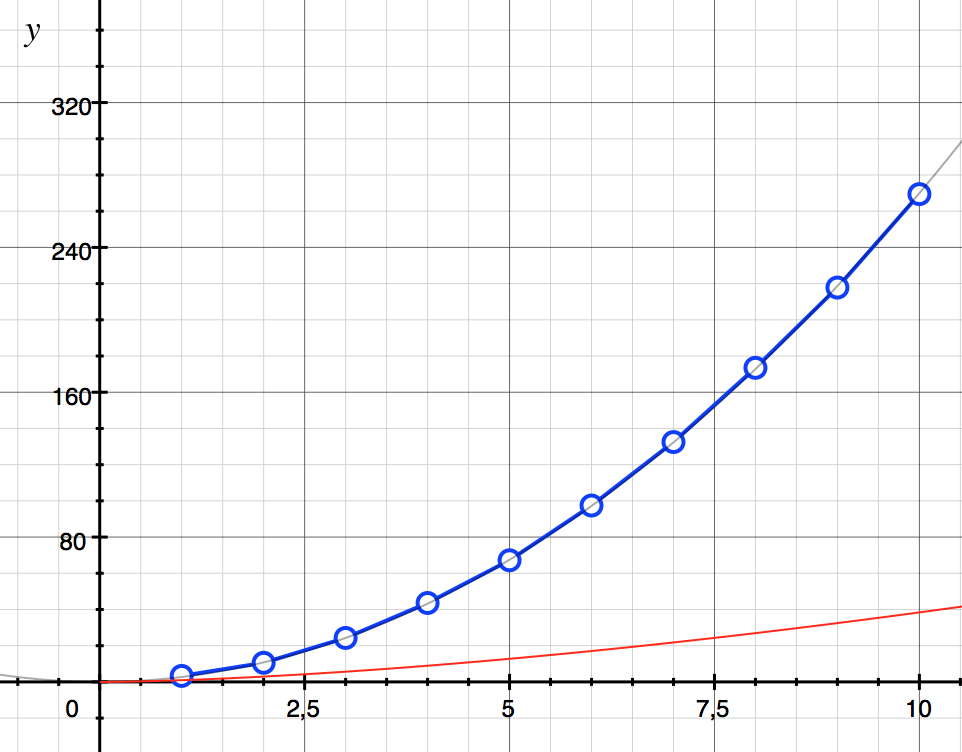# 3rd-grade & Karatsuba multiplication Algorithms

In this post we’re going to study the third-grade algorithm to compute the product of two numbers, and we’re going to compare it with a much more efficient algorithm: The Karatsuba multiplication algorithm.

Did you know that these two algorithms are the ones used in the built in Python multiplication?

We will talk about the Order of both algorithms and give you Python implementations of both of them.

Maybe you’re thinking, why is she writing now about algorithms? Some time ago, I took the course Algorithms: Design and Analysis, Part 1&2, by Tim Roughgarden,  and it was a lot of fun.

Now I’m taking the course again, but I’m spending more time to review the algorithms and play with them. I really encourage you to take the course if you have some time. But first, read this post 🙂

This is the outline:

• Python code for the third grade product algorithm
• The Karatsuba Algorithm
• Python code for the Karatsuba algorithm

Let’s start! 🙂

First, we’re going to review the third grade algorithm, which all of you already know 🙂

Let’s count the number of operations performed in this step:

– If n is the number of digits of the first number, there are n products and at most n sums (carried numbers).

– So in total, you need 2n operations.

If we continue with the product, we have to repeat n times the same operation (where we assume that n is also the number of digits of the second number):

which gives a total of 2n2 operations.

Finally, you need to sum all these numbers,

which takes around n2 operations more.

So in total, the number of operations is ~3n2, which means that it’s quadratic with the input (proportional to n2).

One point I would like to mention about this algorithm is that it’s complete: no matter what x and y you start with, if you perform correctly the algorithm, the algorithm terminates and finds the correct solution.

## Python code for the third grade product algorithm

Here I give you an example of an implementation of the third grade algorithm, where I have included a Counter for the sum and product operations:

Using that algorithm, if you run

where (third_grade_algorithm.py is the name of the file), you will run the previous code and terminate with the Python console open. This way you can call your algorithm function and try:

So it took 20 + 16 = 36 operations to compute the product of these two numbers.

Once we have this code, we can average the number of operations used in the product of n-digit numbers:

In the following figure, we plot the result of the previous experiment when ntimes = 200 samples and nmax = 10 digits:We can see that these points fit the curve f(n) = 2.7 n2.

Note that 2.7 < 3, the proportionality factor we deduced before. This is because we were assuming the worst case scenario, whereas the average product takes into account random numbers.

To sum up, the 3rd grade algorithm is an Ο(n2) complete algorithm.

But can we do better? Is there an algorithm that performs the product of two numbers quicker?

The answer is yes, and in the following section we will study one of them: The Karatsuba Algorithm.

## The Karatsuba Algorithm

Let’s first explain this algorithm through an example:

Imagine you want to compute again the product of these two numbers:

In order to do so, we will first decompose them in a singular way:

and the same for y:

If we want to know the product x * y:

Where we can calculate the following 3 parts separately:

However, we need two compute two products for the B term. Let’s see how we can compute only one product to reduce calculations 🙂

If we expand the product:

we see than the right hand side of the equation contains all A,B and C terms.

In particular, if we isolate B = ad + bc, we get:

Great! Now we only need three smaller products in order to compute x * y:

Let’s put some numbers into the previous expressions:

Yes! the result of 1234 * 5678 = 7006652! 🙂

In the next section, we’ll see the pseudo code for the Karatsuba Algorithm and we’re going to translate it into Python code.

## Python code for the Karatsuba Algorithm

In general though, we don’t have 4-digit numbers but n-digit numbers. In that case, the decomposition to be made is:

which is slightly different if n is even or odd.

In pseudocode, the Karatsuba Algorithm is:

And the order of this algorithm Ο(nlog23) ≈ Ο(n1.585) is (more info).

Let’s write now the Python code 🙂

Which is much simpler than the third-grade algorithm right?

Moreover, if we can compare the number of calculations of this algorithm with respect to the third-grade one:where the red line is the Karatsuba algorithm, and the blue lines the 3rd grade one (see above).

Finally, I want to write here one of the quotes mentioned in the Algorithms course, which might encourage you to find a better solution 🙂

Perhaps the most important principle of the good algorithm designer is to refuse to be content.Aho, Hopcroft and Ullman, The Design and Analysis of computer algorithms, 1974